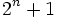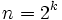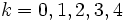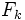Difference between revisions of "Fermat prime"

This article defines a property that can be evaluated for a prime number. In other words, every prime number either satisfies this property or does not satisfy this property.
View other properties of prime numbers | View other properties of natural numbers

Definition

A Fermat prime is a Fermat number that is also a prime number. In other words, it is a prime number of the form, whereis a nonnegative integer.

It turns out that ifis prime fora natural number, thenfor some nonnegative integer. Thus, a Fermat prime can also be defined as a prime of the formfor some natural number.

Occurrence

Finitude conjecture

Further information: Finitude conjecture for Fermat primes

The only known Fermat primes are the Fermat primes for, namely, the primes. For all, either the Fermat primeis known to be composite or its primality is open. The prime number theorem suggests that there are likely to be only finitely many Fermat primes, and it is conjectured that there are no Fermat primes other than the five known ones.

The ID of the sequence in the Online Encyclopedia of Integer Sequences is A019434

Testing

Test for this property

The test used to determine whether a natural number has this property is: Pepin's test

Pepin's test is a deterministic primality test for Fermat numbers. It relies on Proth's theorem, and the observation thatis a quadratic nonresidue for any Fermat prime greater thanitself.